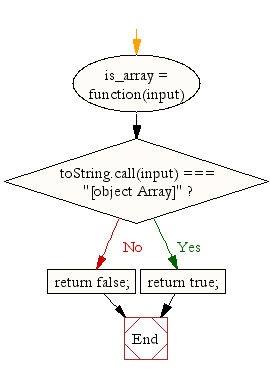# JavaScript: Get the first element of an array

## JavaScript Array: Exercise-1 with Solution

Write a JavaScript function to check whether an 'input' is an array or not.

Test Data:
console.log(is_array('w3resource'));
console.log(is_array([1, 2, 4, 0]));
false
true

Sample Solution:

HTML Code:

``````<!DOCTYPE html>
<html>
<meta charset="utf-8">
<title>JS Bin</title>
<body>
</body>
</html>
```
```

JavaScript Code:

``````var is_array = function(input) {
if (toString.call(input) === "[object Array]")
return true;
return false;
};
console.log(is_array('w3resource'));
console.log(is_array([1, 2, 4, 0]));
```
```

Sample Output:

```false
true
```

Flowchart:ES6 Version:

``````var is_array = input => {
if (toString.call(input) === "[object Array]")
return true;
return false;
};
console.log(is_array('w3resource'));
console.log(is_array([1, 2, 4, 0]));
```
```

Live Demo:

See the Pen JavaScript - Get the first element of an array- array-ex-1 by w3resource (@w3resource) on CodePen.

Improve this sample solution and post your code through Disqus

What is the difficulty level of this exercise?

Test your Programming skills with w3resource's quiz.

﻿

## JavaScript: Tips of the Day

Returns the difference between two arrays, after applying the provided function to each array element of both

Example:

```const tips_differenceBy = (x, y, fn) => {
const s = new Set(y.map(fn));
return x.map(fn).filter(el => !s.has(el));
};

console.log(tips_differenceBy([3.1, 1.5], [3.3, 3.8], Math.floor));
console.log(tips_differenceBy([{ x: 3 }, { x: 2 }], [{ x: 2 }], v => v.x));
```

Output:

```

```